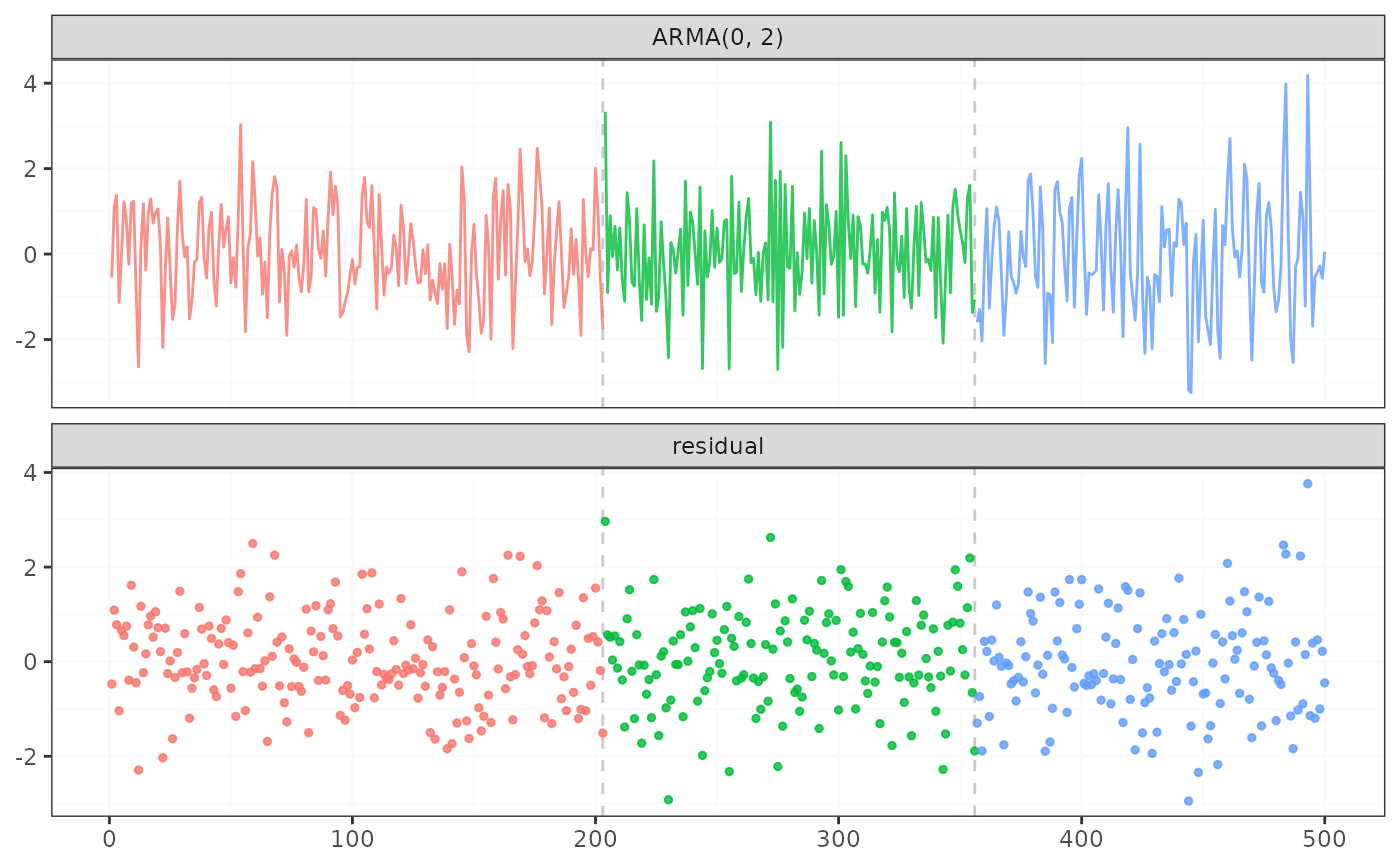fastcpd_ts is a wrapper function for fastcpd to find change points in time series data. The function is similar to fastcpd except that the data is a time series data and the family is one of "ar", "var", "arima" or "garch".

## Usage

fastcpd.ts(data, family = NULL, order = c(0, 0, 0), ...)

fastcpd_ts(data, family = NULL, order = c(0, 0, 0), ...)

## Arguments

data

A numeric vector, a matrix, a data frame or a time series object.

family

A character string specifying the family of the time series. The value should be one of "ar", "var", "arima" or "garch".

order

A positive integer or a vector of length less than four specifying the order of the time series. Possible combinations with family are:

• ar, NUMERIC(1): AR(p) model using linear regression.

• ar, NUMERIC(3): ARIMA(p, 0, 0) model using forecast::Arima, where p is the first element of the vector.

• var, NUMERIC(1): VAR(p) model using linear regression.

• ma, NUMERIC(1): MA(q) model using forecast::Arima.

• ma, NUMERIC(3): ARIMA(0, 0, q) model using forecast::Arima, where q is the third element of the vector.

• arima, NUMERIC(3): ARIMA(p, d, q) model using forecast::Arima.

• garch, NUMERIC(2): GARCH(p, q) model using tseries::garch.

...

Other arguments passed to fastcpd, for example, segment_count. One special argument can be passed here is include.mean, which is a logical value indicating whether the mean should be included in the model. The default value is TRUE.

## Value

A class fastcpd object.

## Examples

# \donttest{
set.seed(1)
n <- 600
x <- rep(0, n + 1)
for (i in 1:300) {
x[i + 1] <- 0.8 * x[i] + rnorm(1, 0, 2)
}
for (i in 301:n) {
x[i + 1] <- 0.1 * x[i] + rnorm(1, 0, 2)
}
result <- fastcpd.ts(
x[1 + seq_len(n)],
"ar",
c(1, 0, 0),
include.mean = FALSE,
trim = 0,
beta = (1 + 1 + 1) * log(n) / 2 * 3
)
summary(result)
#>
#> Call:
#> fastcpd.ts(data = x[1 + seq_len(n)], family = "ar", order = c(1,
#>     0, 0), include.mean = FALSE, trim = 0, beta = (1 + 1 + 1) *
#>     log(n)/2 * 3)
#>
#> Change points:
#> 301
#>
#> Cost values:
#> 623.7791 643.8769
plot(result)# }